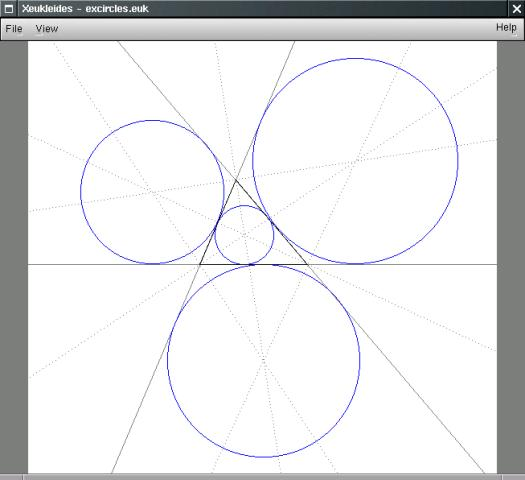## 57 Search Results

The package ADOL-C facilitates the evaluation of first and higher derivatives of vector functions that are defined by computer programs written in C or C++. The resulting derivative evaluation routines may be called from C/C++, Fortran, or any other language that can be linked with C. The numerical values of derivative vectors are obtained free of truncation errors at a small multiple of the run time and randomly accessed memory of the given function evaluation program.

### Axiom

Axiom is a general purpose Computer Algebra system. It is useful for research and development of mathematical algorithms. It defines a strongly typed, mathematically correct type hierarchy. It has a programming language and a built-in compiler.

### CASA

CASA is a special-purpose system for computational algebra and constructive algebraic geometry. The system has been developed since 1990. CASA is the ongoing product of the Computer Algebra Group at the Research Institute for Symbolic Computation (RISC-Linz), the University of Linz, Austria, under the direction of Prof. Winkler. The system is built on the kernel of the widely used computer algebra system Maple.

### CGAL

CGAL is a collaborative effort of several sites in Europe and Israel. The goal is to make the most important of the solutions and methods developed in computational geometry available to users in industry and academia in a C++ library. The goal is to provide easy access to useful, reliable geometric algorithms The CGAL library contains: the Kernel with geometric primitives such as points, vectors, lines, predicates for testing things such as relative positions of points, and operations such as intersections and distance calculation, the Basic Library which is a collection of standard data structures and geometric algorithms, such as convex hull in 2D/3D, (Delaunay) triangulation in 2D/3D, planar map, polyhedron, smallest enclosing circle, and multidimensional query structures, the Support Library which offers interfaces to other packages, e.g., for visualisation, and I/O, and other support facilities.

### Cinderella

Cinderella is a software system for doing geometry on a computer. The new version Cinderella.2 also includes physics simulations and algorithmic elements.

### CoCoA

CoCoA is a system for Computations in Commutative Algebra. It is able to perform simple and sophisticated operations on multivaraiate polynomials and on various data related to them (ideals, modules, matrices, rational functions). For example, it can readily compute Grobner bases, syzygies and minimal free resolution, intersection, division, the radical of an ideal, the ideal of zero-dimensional schemes, Poincare' series and Hilbert functions, factorization of polynomials, toric ideals. The capabilities of CoCoA and the flexibility of its use are further enhanced by the dedicated high-level programming language. For convenience, the system offers a textual interface, an Emacs mode, and a graphical user interface common to most platforms.

### EViews

Eviews supports general statistical analysis and econometric analyses (cross-section, panel data analysis, time series estimation and forecasting). It combines spreadsheet and relational database technology with the traditional tasks found in statistical software. Among its numerous data formats are Excel, databases, PSPP/SPSS, DAP/SAS, Stata, RATS, and TSP. Compatible: numerous formats, including databank format, Excel formats, PSPP/SPSS, DAP/SAS, Stata, RATS, and TSP

### Fast Artificial Neural Network Library

Fast Artificial Neural Network Library is a neural network library that implements multilayer artificial neural networks in C with support for both fully connected and sparsely connected networks. Cross-platform execution in both fixed and floating point are supported. It includes a framework for easy handling of training data sets. It is easy to use, versatile, well documented, and fast. PHP, C++, .NET, Python, Delphi, Octave, Ruby, Pure Data, and Mathematica bindings are available. A reference manual accompanies the library with examples and recommendations on how to use the library. A graphical user interface is also available for the library.

### FLASH Code

The FLASH code is a modular, adaptive, parallel simulation code capable of handling general compressible flow problems in astrophysical environments. It has been designed to allow users to configure initial and boundary conditions, change algorithms, and add new physical effects with minimal effort. It uses the PARAMESH library to manage a block-structured adaptive grid, placing resolution elements only where they are needed most. It uses the Message-Passing Interface (MPI) library to achieve portability and scalability on a variety of different message-passing parallel computers.

### G+Smo

G+Smo (Geometry + Simulation Modules, pronounced "gismo") is a new open-source C++ library that brings together mathematical tools for geometric design and numerical simulation. It is developed mainly by researchers and PhD students. It implements the relatively new paradigm of isogeometric analysis, which suggests the use of a unified framework in the design and analysis pipeline. G+Smo is an object-oriented, cross-platform, template C++ library and follows the generic programming principle, with a focus on both efficiency and ease of use. The library is partitioned into smaller entities, called modules. Examples of available modules include the dimension-independent NURBS module, the data fitting and solid segmentation module, the PDE discretization module and the adaptive spline module, based on hierarchical splines of arbitrary dimension and polynomial degree. The library is licenced under the Mozilla Public License v2.0. It has been developed within the homonym research network supported by the Austrian Science Fund and aims at providing access to high quality, open-source software to the forming isogeometric numerical simulation community and beyond.

More information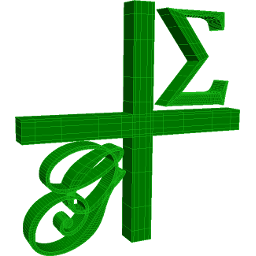### GAP

GAP is a system for computational discrete algebra, with particular emphasis on Computational Group Theory. GAP provides a programming language, a library of thousands of functions implementing algebraic algorithms written in the GAP language as well as large data libraries of algebraic objects. GAP is used in research and teaching for studying groups and their representations, rings, vector spaces, algebras, combinatorial structures, and more. GAP is developed by international cooperation. The system, including source, is distributed freely under the terms of the GNU General Public License. You can study and easily modify or extend GAP for your special use. The current version is GAP 4, the older version GAP 3 is still available.

### Gauss

Introduction: Gauss is an easy-to-use data analysis, mathematical and statistical environment based on the powerful, fast and efficient GAUSS Matrix Programming Language. It is used to solve problems of exceptionally large scale. Program development and program execution are fast. Programs in command line mode (as in DOS or Unix); a limited Windows graphical user interface. GAUSS plot features a fully functional, interactive GUI. It can be used as a tool to design their own algorithms, for doing quick simulations, to write compact programs given the number of matrix-based statistical and financial functions, to used for numerical computation, and handle matrices in the same way as scalars. It provides a C-library interface.

### GeoGebra

GeoGebra is free and multi-platform dynamic mathematics software for all levels of education that joins geometry, algebra, tables, graphing, statistics and calculus in one easy-to-use package. It has received several educational software awards in Europe and the USA.Quick Facts: * Graphics, algebra and tables are connected and fully dynamic * Easy-to-use interface, yet many powerful features * Authoring tool to create interactive learning materials as web pages * Available in many languages for our millions of users around the world * Free and open source software

More information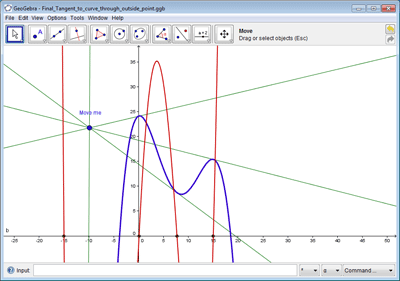### Global Optimization Toolbox For Maple

Optimization is the science of finding solutions that satisfy complicated constraints and objectives. In engineering, constraints may arise from technical issues. In business, constraints are related to many factors, including cost, time, and staff. The objective of global optimization is to find [numerically] the absolute best solution of highly nonlinear optimization models that may have a number of locally optimal solutions. Global optimization problems can be extremely difficult. Frequently engineers and researchers are forced to settle for solutions that are “good enough” at the expense of extra time, money, and resources, because the best solution has not been found. Using the Global Optimization Toolbox, you can formulate your optimization model easily inside the powerful Maple numeric and symbolic system, and then use world-class Maple numeric solvers to return the best answer, fast! Illustrative references: 1. Pintér, J. D. Global Optimization in Action. Springer Science, 1996, 512 p., ISBN: 978-0-7923-3757-7 Winner of the 2000 INFORMS Computing Society Prize. 2. Pintér, J. D., Linder, D. and Chin, P. Global Optimization Toolbox for Maple: An introduction with illustrative applications. Optimization Methods and Software 21 (2006) (4) 565-582.

### gretl - Gnu Regression, Econometrics and Time-series Library

Gretl is a statistical package. It was developed mainly for econometrics. It has as well scripting capabilities as an easy intuitive interface. It features a wide variety of estimators, time series methods limited dependent variables. It has several times been applied in the Journal of Applied Econometrics.

### IBM SPSS Statistics

With IBM SPSS Statistics, you can get a quick look at your data, formulate hypotheses for additional testing, and then carry out a number of procedures to help clarify relationships between variables, create clusters, identify trends and make predictions.

### igraph

igraph is a collection of network analysis tools with the emphasis on efficiency, portability and ease of use. igraph is a free and open source software package for creating and manipulating undirected and directed graphs. It includes implementations for classic graph theory problems like minimum spanning trees and network flow, and also implements algorithms for some recent network analysis methods, like community structure search. The efficient implementation of igraph allows it to handle graphs with millions of vertices and edges. The rule of thumb is that if your graph fits into the physical memory then igraph can handle it. igraph can be programmed in R, Python and C/C++ by virtue of R/igraph, python-igraph and C/igraph, respectively. There is also a Mathematica interface IGraph/M written by Szabolcs Horvát.

### ILOG CPLEX

ILOG CPLEX is an environment for optimization problems. ILOG CPLEX algorithms can be accessed from the CPLEX Component Libraries as well as the CPLEX Interactive Optimizer, an easy-to-use interactive program. CPLEX provides all the basic features and utilities for using these solvers: sophisticated problem preprocessing; file reading and writing utilities; reporting; messaging control; interactive revision capability; efficient restart from an advanced basis; sensitivity analysis; and an infeasibility finder.

### IML - Integer Matrix Library

IML is a free library of C source code which implements algorithms for computing exact solutions to dense systems of linear equations over the integers. IML is designed to be used with the ATLAS/BLAS library and GMP bignum library. Written in portable C, IML can be used on both 32-bit and 64-bit machines. It can be called from C++.

### KANT

KASH/KANT is a computer algebra system for sophisticated computations in algebraic number fields and global function fields. It has been developed under the project leadership of Prof. Dr. M. Pohst at Technische Universität Berlin.

### Kenzo

Kenzo is a program for effective algebraic topology

### KnotPlot

KnotPlot is a program to visualize and manipulate knots in three and four dimensions. Knots can be loaded from a database of almost 1000 knots and links or sketched by hand in three dimensions. Also, knots may be constructed via the Conway notation or using a tangle calculator. A number of special knot types (torus knots, knot chains, Lissajous knots) may be created on the fly. Finally, new knots can be created from old knots using a number of transformations.

### LAPACK

LAPACK is written in Fortran77 and provides routines for solving systems of simultaneous linear equations, least-squares solutions of linear systems of equations, eigenvalue problems, and singular value problems. The associated matrix factorizations (LU, Cholesky, QR, SVD, Schur, generalized Schur) are also provided, as are related computations such as reordering of the Schur factorizations and estimating condition numbers. Dense and banded matrices are handled, but not general sparse matrices. In all areas, similar functionality is provided for real and complex matrices, in both single and double precision.

### M4RI

M4RI is a library for fast arithmetic with dense matrices over F2.

More information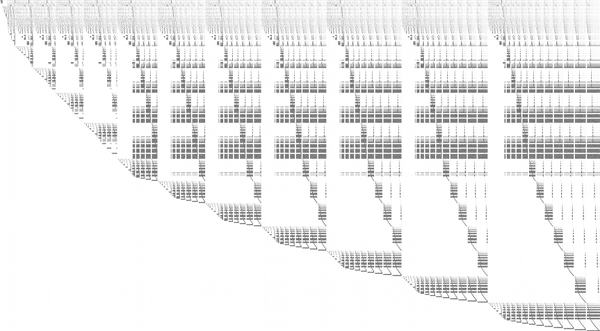### Magma

Magma is a large, well-supported software package designed to solve computationally hard problems in algebra, number theory, geometry and combinatorics. It provides a mathematically rigorous environment for computing with algebraic, number-theoretic, combinatoric and geometric objects.

### Maple

Maple is an environment for scientific and engineering problem-solving, mathematical exploration, data visualization and technical authoring.

More information### Mathematica

Mathematica seamlessly integrates a numeric and symbolic computational engine, graphics system, programming language, documentation system, and advanced connectivity to other applications.

### Matlab

MATLAB is a high-level language and interactive environment that enables you to perform computationally intensive tasks faster than with traditional programming languages such as C, C++, and Fortran.

### Maxima

Maxima is a system for the manipulation of symbolic and numerical expressions, including differentiation, integration, Taylor series, Laplace transforms, ordinary differential equations, systems of linear equations, and vectors, matrices, and tensors. Maxima produces high precision results by using exact fractions and arbitrarily long floating point representations, and can plot functions and data in two and three dimensions.

### nauty

Nauty is a program for computing automorphism groups of graphs and digraphs. It can also produce a canonical labelling.

### NetworkX

NetworkX (NX) is a Python package for the creation, manipulation, and study of the structure, dynamics, and functions of complex networks.

### Normaliz

Normaliz is a tool for computations in affine monoids, vector configurations, lattice polytopes, and rational cones. Its input data can be specified in terms of a system of generators or vertices or a system of linear homogeneous Diophantine equations, inequalities and congruences or a binomial ideal. Normaliz computes the dual cone of a rational cone (in other words, given generators, Normaliz computes the defining hyperplanes, and vice versa), a placing (or lexicographic) triangulation of a vector configuration (resulting in a triangulation of the cone generated by it), the Hilbert basis of a rational cone, the lattice points of a lattice polytope, the normalization of an affine monoid, the Hilbert (or Ehrhart) series and the Hilbert (or Ehrhart) (quasi) polynomial under a Z-grading (for example, for rational polytopes), NEW: generalized (or weighted) Ehrhart series and Lebesgue integrals of polynomials over rational polytopes via NmzIntegrate, a description of the cone and lattice under consideration by a system of inequalities, equations and congruences

### NTL

NTL is a high-performance, portable C++ library providing data structures and algorithms for manipulating signed, arbitrary length integers, and for vectors, matrices, and polynomials over the integers and over finite fields.

### NumXL

NumXL is a suite of time series Excel add-ins. It transforms your Microsoft Excel application into a first-class time series software and econometrics tool, offering the kind of statistical accuracy offered by the leading statistical packages. NumXL integrates natively with Excel, adding scores of econometric functions, a rich set of shortcuts, and intuitive user interfaces to guide you through the entire process.

More information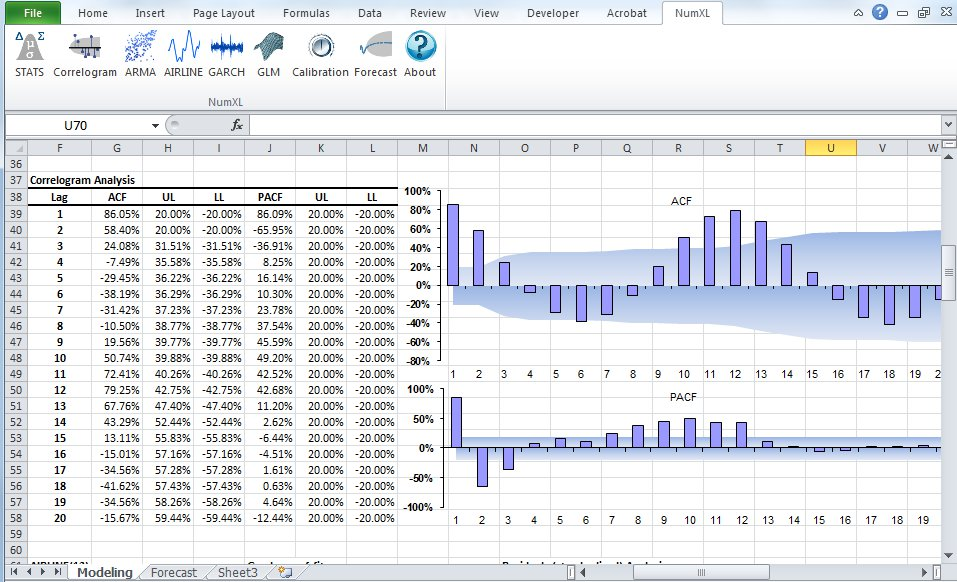### Octave

GNU Octave is a high-level language, primarily intended for numerical computations. It provides a convenient command line interface for solving linear and nonlinear problems numerically, and for performing other numerical experiments using a language that is mostly compatible with Matlab. It may also be used as a batch-oriented language. Octave has extensive tools for solving common numerical linear algebra problems, finding the roots of nonlinear equations, integrating ordinary functions, manipulating polynomials, and integrating ordinary differential and differential-algebraic equations. It is easily extensible and customizable via user-defined functions written in Octave's own language, or using dynamically loaded modules written in C++, C, Fortran, or other languages.

### OxMetrics

The family of software packages for the econometric analysis of time series, forecasting, econometric model selection and for the statistical analysis of cross-section data and panel data.

### PLTMG

PLTMG is a package for solving elliptic partial differential equations in general regions of the plane. It is based on continuous piecewise linear triangular finite elements, and features adaptive local mesh refinement, multigraph iteration, and pseudo-arclength continuation options for parameter dependencies. It also provides options for solving several classes of optimal control and obstacle problems. The package includes an initial mesh generator and several graphics packages. Support for the Bank-Holst parallel adaptive meshing strategy is also provided. PLTMG is provided as Fortran (and a little C) source code, in both single and double precision versions. The code has interfaces to X-Windows, MPI, and Michael Holst's OpenGL image viewer SG. The X-Windows, MPI, and SG interfaces require libraries that are NOT provided as part of the PLTMG package.

### PSPP

PSPP is a program for statistical analysis of sampled data. It is a Free replacement for the proprietary program SPSS.

### QPA = Quivers and Path Algebras

QPA provides software for doing computations over finite dimensional quotients of path algebras. QPA has data structures for quivers, quotients of path algebras, and modules, homomorphisms and complexes of modules over quotients of path algebras. It has implementations for computing homomorphism spaces, projective resolutions, extension groups, generators of Ext-algebras, almost split sequences and more.

### R

R is a language and environment for statistical computing and graphics. It is a GNU project which is similar to the S language and environment which was developed at Bell Laboratories (formerly AT&T, now Lucent Technologies) by John Chambers and colleagues. R can be considered as a different implementation of S. There are some important differences, but much code written for S runs unaltered under R. R provides a wide variety of statistical (linear and nonlinear modelling, classical statistical tests, time-series analysis, classification, clustering, ...) and graphical techniques, and is highly extensible. The S language is often the vehicle of choice for research in statistical methodology, and R provides an Open Source route to participation in that activity. One of R's strengths is the ease with which well-designed publication-quality plots can be produced, including mathematical symbols and formulae where needed. Great care has been taken over the defaults for the minor design choices in graphics, but the user retains full control.

### RATS

RATS (Regression Analysis of Time Series) is a fast, efficient, and comprehensive econometrics and time series analysis software package.

### Reduce

REDUCE is an interactive system for general algebraic computations of interest to mathematicians, scientists and engineers. It has been produced by a collaborative effort involving many contributors. It is often used as an algebraic calculator for problems that are possible to do by hand. However, REDUCE is designed to support calculations that are not feasible by hand. Many such calculations take a significant time to set up and can run for minutes, hours or even days on the most powerful computers.

### Risa/Asir

Risa/Asir is a general computer algebra system and also a tool for various computation in mathematics and engineering. The development of Risa/Asir started in 1989 at FUJITSU. Binaries have been freely available since 1994 and now the source code is also free. Currently Kobe distribution is the most active branch of its development. We characterize Risa/Asir as follows: (1) An environment for large scale and efficient polynomial computation. (2) A platform for parallel and distributed computation based on OpenXM protocols.

### Sage

SAGE is a framework for number theory, algebra, and geometry computation. It is open source and freely available under the terms of the GNU General Public License (GPL). SAGE is a Python library with a customized interpreter. It is written in Python, C++, and C (via Pyrex). Python (http://www.python.org) is an open source object-oriented interpreted language, with a large number of libraries, e.g., for numerical analysis, which are available to users of SAGE. Python can also be accessed in library mode from C/C++ programs. SAGE provides an interface to several important open source libraries, including Cremona’s MWRANK library for computing with elliptic curves, the PARI library (pari.math.u-bordeaux.fr) for number theory, Shoup’s number theory library NTL (http://www.shoup.net/ntl/), SINGULAR (http://www.singular.uni-kl.de) for commutative algebra, GAP (http://www.gap-system.org) for group theory and combinatorics, and maxima (http://maxima.sourceforge.net) for symbolic computation and calculus.

More information### Scilab

Scilab is a numerical computation system similiar to Matlab or Simulink. Scilab includes hundreds of mathematical functions, and programs from various languages (such as C or Fortran) can be added interactively. It has sophisticated data structures (including lists, polynomials, rational functions, and linear systems), an interpreter, and a high-level programming language. Scilab has been designed to be an open system where the user can define new data types and operations on these data types by using overloading. A number of toolboxes are available with the system.

### Scilab Image Processing Toolbox

SIP provides image processing, pattern recognition, and computer vision routines for SciLab, a Matlab-like matrix-oriented programming environment. SIP is able to read/write images in almost 90 major formats, including JPEG, PNG, BMP, GIF, FITS, and TIFF. It includes routines for filtering, segmentation, edge detection, morphology, curvature, fractal dimension, distance transforms, multiscale skeletons, and more.

### SINGULAR

SINGULAR is a Computer Algebra system for polynomial computations in commutative algebra, algebraic geometry, and singularity theory. SINGULAR's main computational objects are ideals and modules over a large variety of baserings. The baserings are polynomial rings over a field (e.g., finite fields, the rationals, floats, algebraic extensions, transcendental extensions), or localizations thereof, or quotient rings with respect to an ideal. SINGULAR features fast and general implementations for computing Groebner and standard bases, including e.g. Buchberger's algorithm and Mora's Tangent Cone algorithm. Furthermore, it provides polynomial factorizations, resultant, characteristic set and gcd computations, syzygy and free-resolution computations, and many more related functionalities. Based on an easy-to-use interactive shell and a C-like programming language, SINGULAR's internal functionality is augmented and user-extendible by libraries written in the SINGULAR programming language. A general and efficient implementation of communication links allows SINGULAR to make its functionality available to other programs.

### SOFA

SOFA - Statistics Open For All The user-friendly, open-source statistics, analysis, and reporting package.

### STATISTICA

STATISTICA provides a comprehensive array of data analysis, data management, data visualization, and data mining procedures. Its techniques include a wide selection of predictive modeling, clustering, classification, and exploratory techniques in one software platform.

### Sums over integral points of a polygon

Maple program for computing the sum of values of a polynomial function over the set of integral points of a polygon and the corresponding weighted Ehrhart quasi-polynomial.

### SuperLU

SuperLU is a general purpose library for the direct solution of large, sparse, nonsymmetric systems of linear equations on high performance machines. The library is written in C and is callable from either C or Fortran. The library routines will perform an LU decomposition with partial pivoting and triangular system solves through forward and back substitution. The LU factorization routines can handle non-square matrices but the triangular solves are performed only for square matrices. The matrix columns may be preordered (before factorization) either through library or user supplied routines. This preordering for sparsity is completely separate from the factorization. Working precision iterative refinement subroutines are provided for improved backward stability. Routines are also provided to equilibrate the system, estimate the condition number, calculate the relative backward error, and estimate error bounds for the refined solutions.

### Surfer

Surfer is a programme to visualize real algebraic surfaces in an intuitive and fast way

More information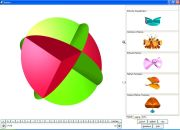### SYMMETRICA

Symmetrica is a program developed by Lehrstuhl Mathematik II of the University of Bayreuth. It has routines to handle the following topics: ordinary representation theory of the symmetric group and related groups, ordinary representation theory of the classical groups, modular representation theory of the symmetric group, projective representation theory of the symmetric group, combinatorics of tableaux, symmetric functions and polynomials, commutative and non commutative Schubert polynomials, operations of finite groups, ordinary representation theory of Hecke algebras of type An

### SymPy

SymPy is a Python library for symbolic mathematics. It aims to become a full-featured computer algebra system (CAS) while keeping the code as simple as possible in order to be comprehensible and easily extensible. SymPy is written entirely in Python and does not require any external libraries.

### Theorema

The Theorema project aims at extending current computer algebra systems by facilities for supporting mathematical proving. The present early-prototype version of the Theorema software system is implemented in Mathematica . The system consists of a general higher-order predicate logic prover and a collection of special provers that call each other depending on the particular proof situations. The individual provers imitate the proof style of human mathematicians and produce human-readable proofs in natural language presented in nested cells. The special provers are intimately connected with the functors that build up the various mathematical domains. The long-term goal of the project is to produce a complete system which supports the mathematician in creating interactive textbooks, i.e. books containing, besides the ordinary passive text, active text representing algorithms in executable format, as well as proofs which can be studied at various levels of detail, and whose routine parts can be automatically generated. This system will provide a uniform (logic and software) framework in which a working mathematician, without leaving the system, can get computer-support while looping through all phases of the mathematical problem solving cycle.

More information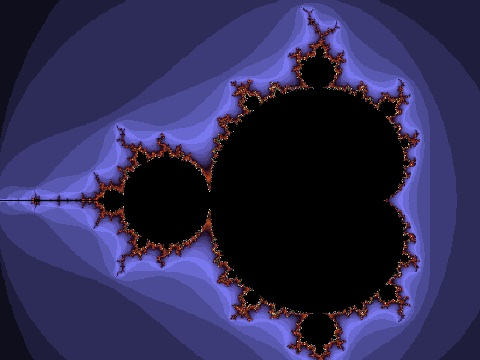More information